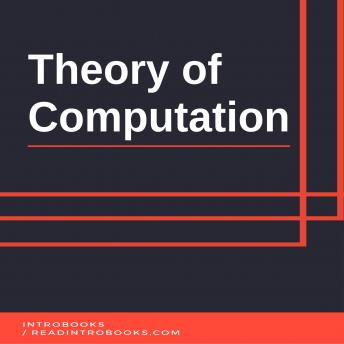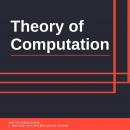# Theory of Computation

#### Summary:

Theory of computation is seen as a branch of both theoretical computer science and modern mathematics (however, it also contains some concepts from pure mathematics). Theory of computation shows how one can effectively solve a problem using a computational model. A number of computational models are described in theory of computation. Algorithm is most common format of computational model. Algorithm is a logical, systematic presentation of the process of problem solution. It theoretically represents the procedure of solving a particular problem. Flowchart is another form of such model of computation. Simply, flowchart is a graphical representation of any algorithm, using various symbols. Each symbol of flowchart represents a particular action. Algorithms and flowcharts possess a strong relation among each other. Yet, theory of computation talks more deeply and descriptively about algorithms and less about flowcharts.

Theories, which are too broad, are often divided into sub theories or branches. Such division allows one to approach and learn the theory efficiently. Theory of computation is divided into four main branches. Many people see these branches as individual sub theories (sub theories and branches are both different terms). These main branches/ sub theories are as following:

Automata Theory Formal Language TheoryComputability TheoryComputational Complexity TheoryHere, it should be noticed that many theories treat Automata Theory and Formal Language Theory as individual concepts. It is same for vice versa situation. Many treat them as a whole. In this book, they are described as individual concepts. However, you will find many connections between their concepts. The whole structure of computation theory is dedicated to describe the capabilities and limitations of modern computer system; in terms of solving a particular problem, (This process includes various steps and operations).

Model of computations are mathematical abstractions of computers and their functionality. These models are used to descriptive studies and researches related to computers and various functions provided by them (capabilities, limitations, etc). These computational models are divided in various types according to the characteristics and results they provide. Turning machine is one of these many models of computations. This model is being referred here since it is arguably the most reasonable and powerful model compared to other computational models. This model has a simple and straightforward structure that is easy to interpret and understand which makes the formulation and analyzing process much easier. Other models consider that infinite memory capacity is essential in order to provide results related to decidable problems. However, turning machine requires only a finite amount of memory in order to solve and provide results for a decidable problem. It means that if a turning model is capable of solving a problem; the very same problem can be solved using any other computer that has a finite amount of available memory.

However, some researchers denied the turning model being the most reliable model. They do not deny its functions or capabilities, but they do point out some limitations ignoring the fact that they are yet to be proven practically.

## Theory of Computation

#### by Introbooks##### Enter your email below to be notified as soon as it is available!

By clicking "Notify Me" you consent to receiving electronic marketing communications from Audiobooks.com. You will be able to unsubscribe at any time.